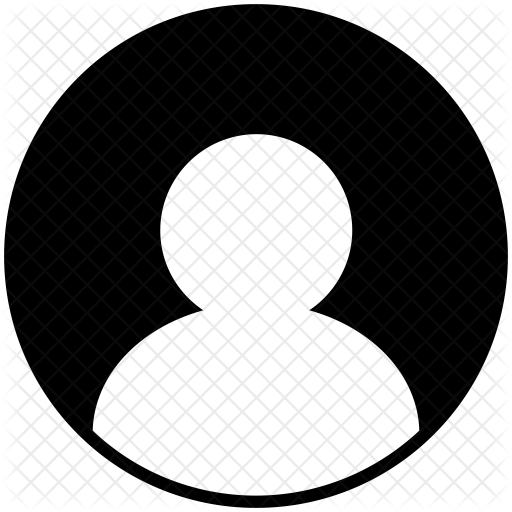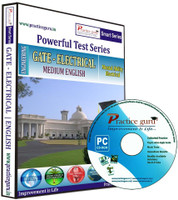﻿ PRACTICE GURU GATE - ELECTRICAL ENGINEERING - PRICE 1125 18 % OFF #EDUCRATSWEB
Guest Post | Submit   Job information   Contents   Link   Youtube Video   Photo   Practice Set   Affiliated LinkRegisterLogin### Practice Guru GATE - Electrical Engineering

Published on offer from flipkart dated Monday April 30 2018 by Neha SharmaPractice Guru GATE - Electrical Engineering Price 1375 1125 18 % Off

Engineering Mathematics:Linear Algebra: Matrix Algebra, Systems of linear equations, Eigen values and Eigen vectors Calculus: Mean value theorems, Theorems of integral calculus, Evaluation of definite and improper integrals, Partial Derivatives, Maxima and minima, Multiple integrals, Fourier series Vector identities, Directional derivatives, Line, Surface and Volume integrals, Stokes, Gauss and Green's theoremsDifferential equations:First order equation (linear and nonlinear), Higher order linear differential equations with constant coefficients, Method of variation of parameters, Cauchy's and Euler's equations, Initial and boundary value problems, Partial Differential Equations and variable separable method.Complex variables:Analytic functions, Cauchy's integral theorem and integral formula, Taylor's and Laurent' series, Residue theorem, Solution integrals.Probability and Statistics:Sampling theorems, Conditional probability, Mean, Median, Mode and standard deviation, Random variables, Discrete and continuous distributions, Poisson, Normal and Binomial distribution, Correlation and regression analysis.Numerical Methods:Solutions of non-linear algebraic equations, Single and multi-step methods for differential equations,Transform Theory:Fourier transform, Laplace transform, Z-transform Electrical Engineering: Electric Circuits and Fields:Network graph, KCL, KVL, Node and mesh analysis, Transient response of dc and ac networks; sinusoidal steady-state analysis, Resonance, Basic filter concepts; ideal current and voltage sources, The venin?s, Norton's and Superposition and Maximum Power Transfer theorems, Two-port networks, Three phase circuits; Gauss Theorem, Electric field and potential due to point, Line, Plane and spherical charge distributions; Ampere's and Biot-Savart's laws; inductance; dielectrics; capacitance.Signals and Systems:Representation of continuous and discrete-time signals; shifting and scaling operations; linear, time-invariant and causal systems; Fourier series representation of continuous periodic signals; sampling theorem; Fourier, Laplace and Z transformsElectrical Machines:Single phase transformer -equivalent circuit, Pastor diagram, Tests, Regulation and efficiency; three phase transformers-connections, Parallel operation, Auto-transformer, Energy conversion principles, DC machines ?types, Windings, Generator characteristics, Armature reaction and commutation, Starting and speed control of motors; three phase induction motors ?principles, Types performance, characteristics, Starting and speed control; single phase induction motors; synchronous machines ?performance, Regulation and parallel operation of generators, Motor starting, Characteristics and applications; servo and stepper motors.Power Systems:Basic power generation concepts; transmission line models and performance; cable performanceinsulation; corona and radio interference; distribution systems; per-unit quantities; bus impedance and admittance matrices; load flow; voltage control; power factor correction; economic operation; symmetrical components; fault analysis; principles of over-current differential and distance protection; solid state relays and digital protection; circuit breakers; system stability concepts swing curves and equal area criterion; HVDC transmission and FACTS conceptsControl Systems:Principles of feedback; transfer function; block diagrams; steady-state errors; Rough and Inquest techniques; Bode plots; root loci; lag lead and lead-lag compensation; state space model; state transition matrix, controllability and observe abilityElectrical and Electronic Measurements:Bridges and potentiometers; PMMC, Moving iron, Dynamometer and induction type instruments; measurement of voltage, Current Power energy and power factor; instrument transformers; digital voltmeters and millimeters; phase time and frequency measurement; Q-meters; oscilloscopes; potentiometric recorders; error analysisAnalog and Digital Electronics:Characteristics of diodes, BJT, FET; amplifiers ?biasing equivalent circuit and frequency response; oscillators and feedback amplifiers; operational amplifiers -characteristics and applications; simple active filters; VCOs and timers; combinational and sequential logic circuits; multiplexer; Schmitt trigger; multi-vibrators; sample and hold circuits; A/D and D/A converters; 8-bit microprocessor basics, Architecture, Programming and interfacingPower Electronics and Drives:Semiconductor power diodes, Transistors, thrusters, traces, GTOs, MOSFETs and IGBTs -static characteristics and principles of operation; triggering circuits; phase control rectifiers; bridge converters -fully controlled and half controlled; principles of choppers and inverters; basis concepts of adjustable speed dc and ac drives + MOCK TESTS for GATE - GATE - ElectricalAdditional Features!1. Runs without Internet!2. Immediate scores and solutions!3. Compatible with Windows XP/7/84. Online support5. Complete career planner chart with PDF files containing relevant details.6. Fun games for brain exercise7. Previous year papers in PDF format also, for print out facility!8. Random questions in a test every time you practice!